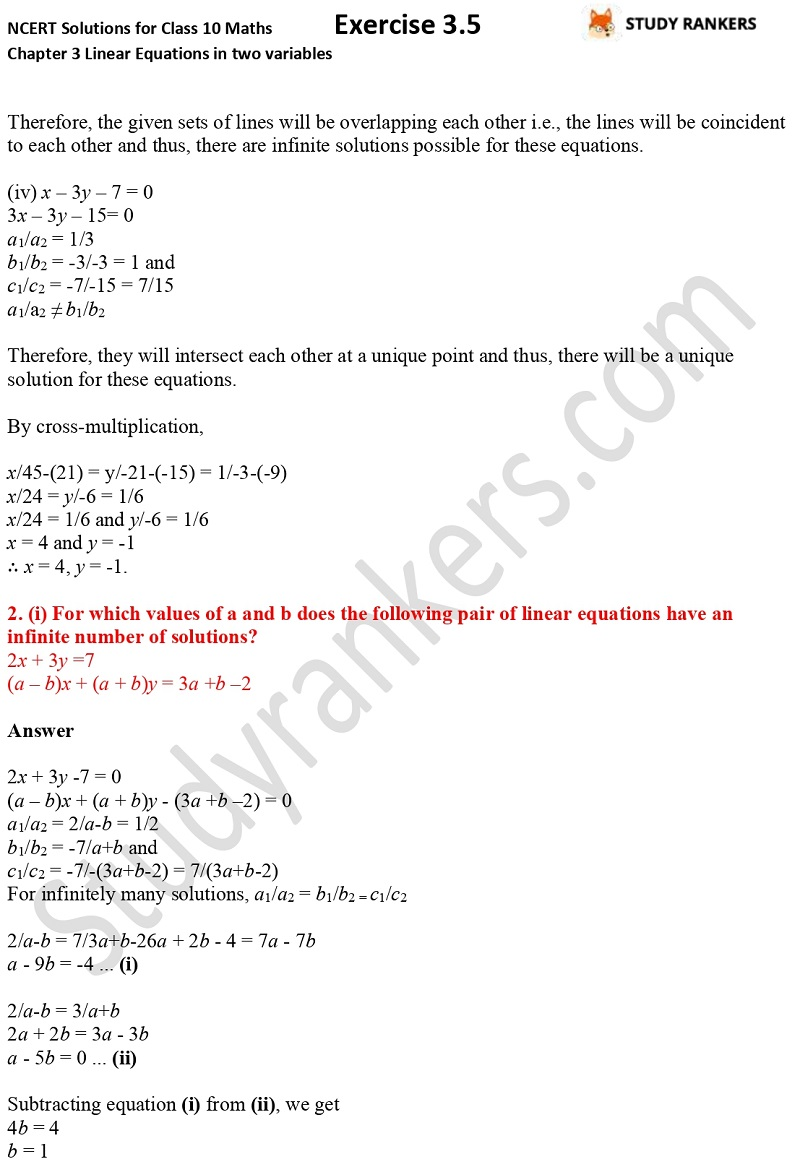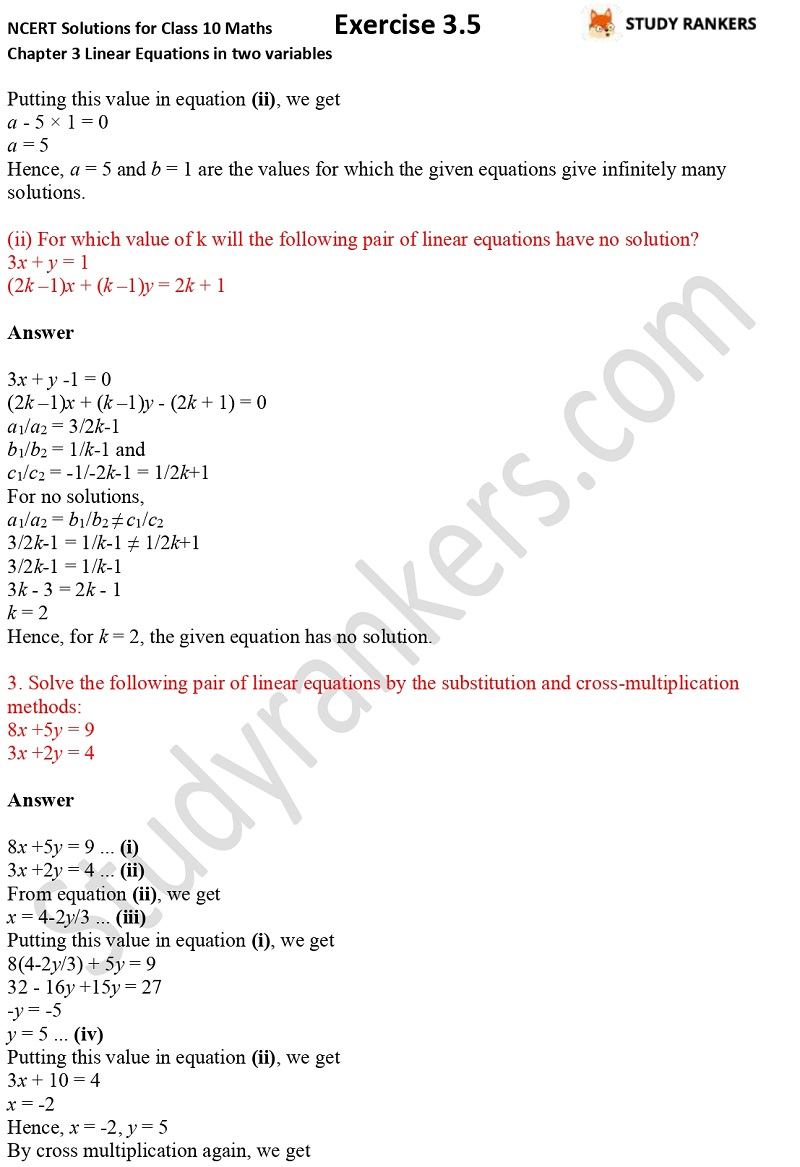## NCERT Solutions for Class 10 Maths Chapter 3 Pair of Linear Equations in Two Variables Exercise 3.5

You can find Chapter 3 Pair of Linear Equations in Two Variables Exercise 3.5 NCERT Solutions for Class 10 Maths that will help you in improving marks in the examination. The solutions are updated as per the latest NCERT Textbooks so you don't have to wander and waste your time. It will increase your problem solving skills. CBSE NCERT Solutions are basic things that a student must complete before going for advance study. There are total four questions in the exercise in which you have to find number of solutions for the pairs of linear equations.